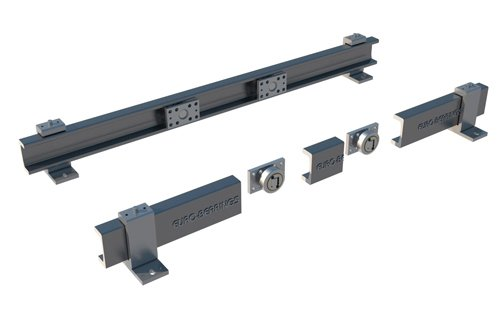# MOMENT-BALANCE CALCULATIONS FOR COMBINED ROLLER BEARINGS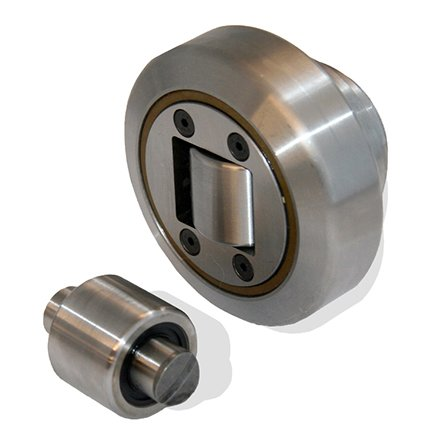## These equations look at the moment-balance of the sliding system in order to calculate the Hertzian Pressure exerted on the steel profiles. In most applications it is the Hertzian Pressure that is the limiting factor. This is the "pressure" at which the point load exerted by the bearings begins to deform the rails (steel profile).

The location of the bearing with respect to the suspension point is critical in determining the force seen by it. The Hertzian static load capacity of the chosen bearing must not be exceeded and this therefore limits the maximum allowable load.

If you need help with any of these calculations, call Kate or Matt (01908 511733) or email a drawing to: sales@euro-bearings.com

### 1. VERTICAL CANTILEVERED APPLICATIONS

Using moment-balance, the bearing distance can be calculated from:

I = (P . L ) / ( 2 . C)

where:

P = Weight of the load being moved (N)

L = Distance between the load and P, the suspension point (mm)

I = Distance of bearings from the suspension point (mm)

C = Maximum allowable static force per bearing, determined by Hertzian Pressure

Alternatively, this formula may be rearranged to calculate the static force generated :

C = (P . L) / (2 . I)

and this value of C can then be compared with those given in the Hertzian Pressure data to determine which bearing is best suited to the loading conditions.

Worked Example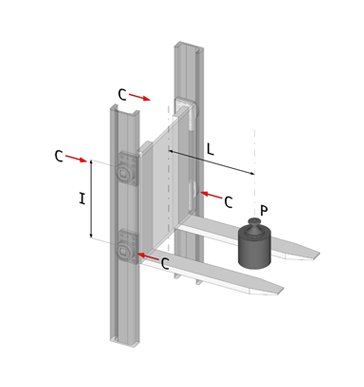### 2. HORIZONTAL CANTILEVERED APPLICATIONS

If your application involves a horizontal telescopic slide then you need the following equations (see diagram below):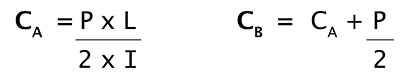Usually CB will be the largest force.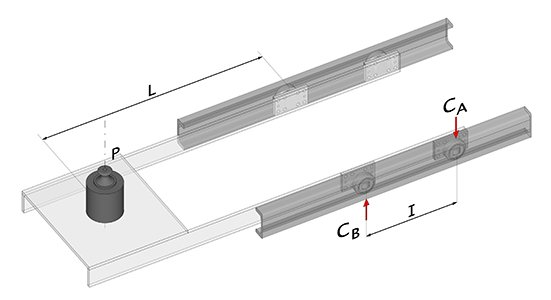### 3. HORIZONTAL APPLICATIONS

In a simple horizontal application there is no need for moment-balance equations. If the load is evenly spread then the load can simply be divided by the number of bearings to calculate the Hertzian Pressure.